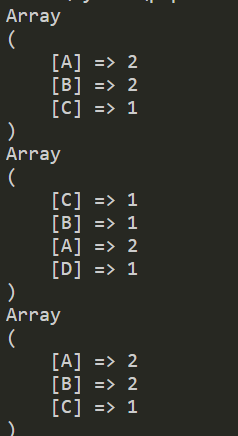# php统计2个数据中同时出现的次数最多的单词

array_count_values 统计数组中值的个数

array_intersect_key 求两个数组的key的交集

<?php

\$arr = array('A', 'B', 'A', 'B', 'C');

\$arr2 = array('C', 'B', 'A', 'D', 'A');

\$arr_count = array_count_values(\$arr);

\$arr2_count = array_count_values(\$arr2);

print_r(\$arr_count);
print_r(\$arr2_count);

\$result = array_intersect_key(\$arr_count, \$arr2_count);

print_r(\$result);